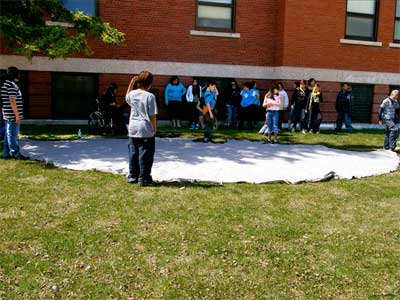SEARCH HOMEMath Central Quandaries & QueriesQuestion from Lacy: Hi there! We are building a tipi for our children. We want to build a large one about 15ft tall with a base of about 15 feet diameter. I am trying to figure out how much canvas we need to accomplish this. I graduated about 20 years ago and am struggling. Please help if you can.Hi Lacy,

Most of the tipis I have seen, like the one in the diagram below, have the canvas in the shape of almost a perfect half circle.Diagram from a tipi raising with elder Glen Anaquod.

I am going to assume you are going to work with a semicircle and then use the formulas that I have in a response to an earlier question. Since the canvas is in the shape of a semicircle $D = 180$ degrees. $H$ is the height of the tipi. $R$ is the radius of the circle at the bottom of the tipi and $P$ is the radius of the semicircle of canvas. ($P$ is also the slant height of the tipi. See the diagrams in this earlier response.)

Since $D = \large \frac{R}{P}$ and $D= 180$ we have that $P = 2 R,$ or $R = \large \frac{P}{2}.$ The other equation is $P = \sqrt{R^2 + H^2}$ and hence $P = \sqrt{\left( \large \frac{P}{2}\right)^2 + H^2}$ or $P^2 = \left( \large \frac{P}{2}\right)^2 + H^2 .$ Hence $P = \large \frac{2}{\sqrt{3}} H.$

You want $H$ to be 15 feet and hence this gives $P = \large \frac{2}{\sqrt{3}} \times 15 = 17.32$ feet. Since $P = 2 R$ this is also the diameter of the base of the tipi.

To make this tipi then you need a semicircle of canvas of radius 17.32 feet.

The base of the tipi is slightly larger than you wanted so let me know if this is not suitable,
PennyMath Central is supported by the University of Regina and The Pacific Institute for the Mathematical Sciences.